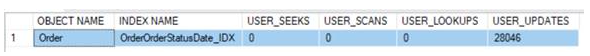# SQL - How to create a computed Column in SQL Server

In this post, we will learn how to create a computed column in SQL and how to define the calculation logic for this computed column.

A computed column is a virtual column that is not physically stored in the table, unless the column is marked PERSISTED. A computed column expression can use data from other columns to calculate a value for the column to which it belongs
Here is an example to calculate the DiscountedAmount for each order

DiscountedAmount = OrderAmount * 25%

Syntax: Create SQL Computed Column on Create Table:

The below SQL script creates a table with Computed Column ‘DiscountedAmount’ and field value will be calculated based on other column

CREATE TABLE Orders (
OrderID int NOT NULL,
OrderNumber int NOT NULL,
StatusID int,
OrderAmount Decimal(10,2),
OrderDate date DEFAULT GETDATE(),
DiscountedAmount AS (OrderAmount * .25),
PRIMARY KEY (OrderID),
);

When you fetch records from table, the Computed field DiscountedAmount will have calculated value based on defined expression (OrderAmount * .25) in schema.

----Inserts value in the table

Insert dbo.Orders(OrderID,OrderNumber,StatusID,OrderAmount)
Select 1, 100, 1, 100

Insert dbo.Orders(OrderID,OrderNumber,StatusID,OrderAmount)
Select 2, 101, 1, 300

----Display the rows in the table

Select * from dbo.Orders

Output:

Syntax: Create SQL Computed Column on Alter Table:

The below SQL script creates a Computed Column ‘DiscountedAmount’ on an existing table

ALTER TABLE dbo.Orders ADD DiscountedAmount AS (OrderAmount * .25)

Syntax: Drop SQL Computed Column:

The below SQL script delete a Computed Column ‘DiscountedAmount’ from table

ALTER TABLE dbo.Orders DROP COLUMN DiscountedAmount

### SQL Server - Identify unused indexes

In this blog, we learn about the index usage information (SYS.DM_DB_INDEX_USAGE_STATS) and analyze the index usage data (USER_SEEKS, USER_S...